GRE Subject Test: Math : Axioms of Probability

Example Questions

Example Question #1 : Axioms Of Probability

A student has 14 piece of gum, 3 are spearmint, 5 are peppermint, and the rest are cinnamon. If one piece of gum is chosen at random, which of the following is NOT true.

The probability of picking a cinnamon or a peppermint is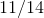.

The probability of picking a cinnamon is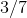.

The probability of picking a spearmint is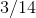.

The probability of picking a spearmint or a cinnamon is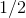.

The probability of not picking a cinnamon is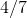.

The probability of picking a spearmint or a cinnamon is.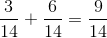not